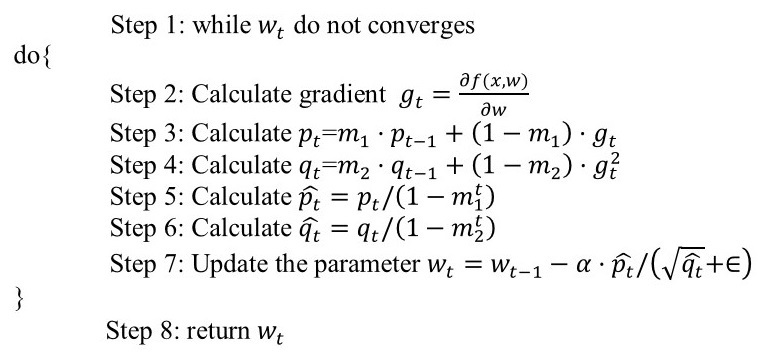Open In App

Prerequisite: Optimization techniques in Gradient Descent

Gradient Descent is applicable in the scenarios where the function is easily differentiable with respect to the parameters used in the network. It is easy to minimize continuous functions than minimizing discrete functions. The weight update is performed after one epoch, where one epoch represents running through an entire dataset. This technique produces satisfactory results but it deteriorates if the training dataset size becomes large and does not converge well. It also may not lead to a global minimum in case of the existence of multiple local minima.

Stochastic gradient descent overcomes this drawback by randomly selecting data samples and updating the parameters based on the cost function. Additionally, it converges faster than regular gradient descent and saves memory by not accumulating the intermediate weights.
Adaptive Moment Estimation (ADAM) facilitates the computation of learning rates for each parameter using the first and second moment of the gradient.

Being computationally efficient, ADAM requires less memory and outperforms on large datasets. It require p2, q2, t to be initialized to 0, where p0 corresponds to 1st moment vector i.e. mean, q0 corresponds to 2nd moment vector i.e. uncentered variance and t represents timestep.
While considering ƒ(w) to be the stochastic objective function with parameters w, proposed values of parameters in ADAM, are as follows:

`α = 0.001, m1=0.9, m2=0.999,  ϵ = 10-8. `

Another major advantage discussed in the study of ADAM is that the updation of the parameter is completely invariant to gradient rescaling, the algorithm will converge even if the objective function changes with time. The drawback of this particular technique is that it requires the computation of second-order derivatives which results in increased cost.

The algorithm of ADAM has been briefly mentioned below –